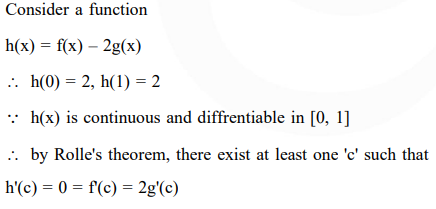# Solve this following`
Question:

If $f$ and $g$ are differentiable functions in $[0,1]$ satisfying $f(0)=2=g(1), g(0)=0$ and $f(1)=6$, then for some $c \in] 0,1[:$

1. $2 f^{\prime}(c)=g^{\prime}(c)$

2. $2 f^{\prime}(c)=3 g^{\prime}(c)$

3. $f^{\prime}(c)=g^{\prime}(c)$

4. $f^{\prime}(c)=2 g^{\prime}(c)$

Correct Option: , 4

Solution: